GeeksforGeeks App
Open AppBrowser
Continue

# Building Naive Bayesian classifier with WEKA

The use of the Naive Bayesian classifier in Weka is demonstrated in this article. The “weather-nominal” data set used in this experiment is available in ARFF format. This paper assumes that the data has been properly preprocessed.

The Bayes’ Theorem is used to build a set of classification algorithms known as Naive Bayes classifiers. It is a family of algorithms that share a common concept, namely that each pair of features being classified is independent of the others.

Steps to be followed:

• Initially, we have to load the required dataset in the weka tool using choose file option. Here we are selecting the weather-nominal dataset to execute.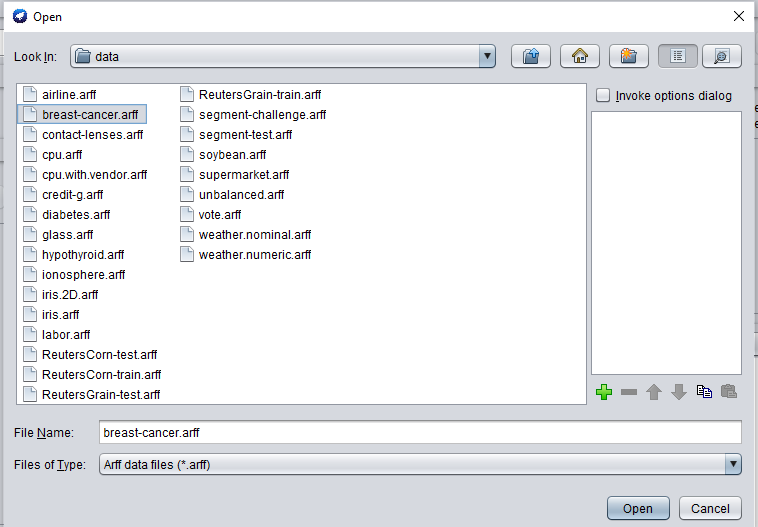• Now we have to go to the classify tab on the top left side and click on the choose button and select the Naive Bayesian algorithm in it.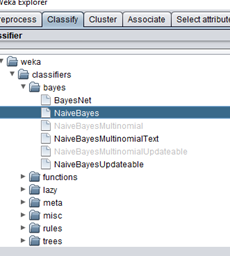• Now to change the parameters click on the right side at the choose button, and we are accepting the default values in this example.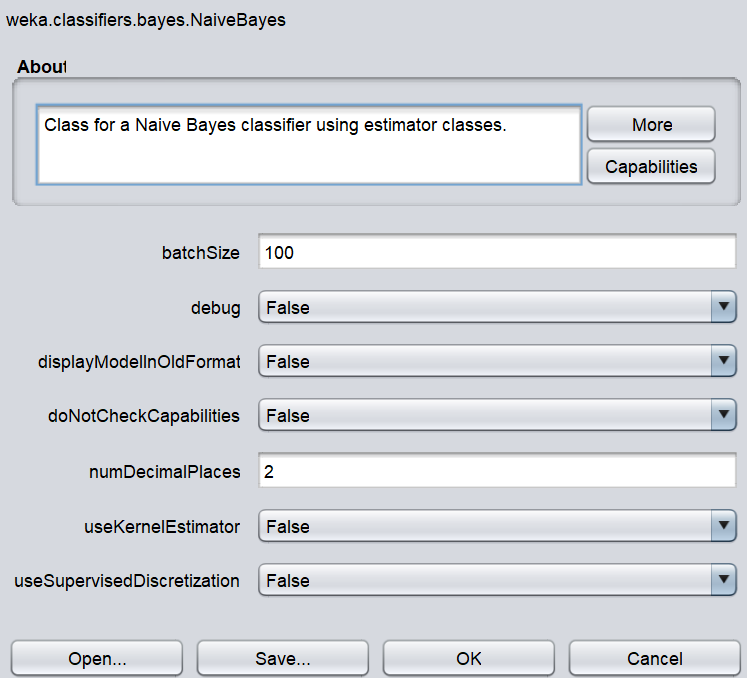• We choose the Percentage split as our measurement method from the “Test” choices in the main panel. Since we don’t have a separate test data collection, we’ll use the percentage split of 66 percent to get a good idea of the model’s accuracy. Our dataset contains 14 examples, with h9 being used for training and 5 being used for testing.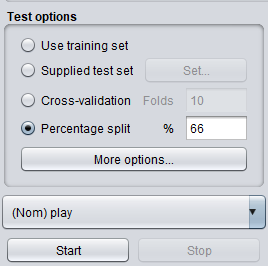• To generate the model, we now click “start.” When the model is done, the evaluation statistic will appear in the right panel.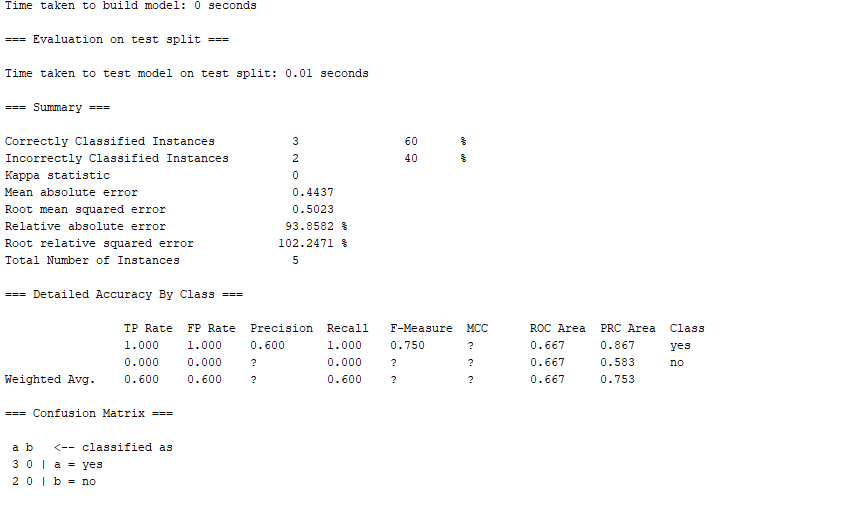The following is the java code for the same

## Java

 `import` `java.io.BufferedReader;``import` `java.io.FileReader;``import` `weka.classifiers.bayes.NaiveBayes;``import` `weka.classifiers.Evaluation;``import` `weka.core.Instances;` `public` `class` `WeatherNominal {``    ``public` `static` `void` `main(String args[]) {``        ``try` `{``            ``// Create naivebayes classifier //``            ``NaiveBayes naivebayes = ``new` `NaiveBayes();``            ` `            ``// Dataset path //``            ``String weatherNominalDataset = ``"/home/droid/Tools/weka-3-8-5/data/weather.nominal.arff"``;``            ``// Create bufferedreader to read the dataset //``            ``BufferedReader bufferedReader = ``new` `BufferedReader(``new` `FileReader(weatherNominalDataset));``            ` `            ``// Create dataset instances //``            ``Instances datasetInstances = ``new` `Instances(bufferedReader);``            ` `            ``// Randomize the dataset //``            ``datasetInstances.randomize(``new` `java.util.Random(``0``));``            ` `            ``// Divide dataset into training and test data //``            ``int` `trainingDataSize = (``int``) Math.round(datasetInstances.numInstances() * ``0.66``);``            ``int` `testDataSize = (``int``) datasetInstances.numInstances() - trainingDataSize;``            ` `            ``// Create training data //``            ``Instances trainingInstances = ``new` `Instances(datasetInstances,``0``,trainingDataSize);``            ``// Create test data //``            ``Instances testInstances = ``new` `Instances(datasetInstances,trainingDataSize,testDataSize);``            ` `            ``// Set Target class //``            ``trainingInstances.setClassIndex(trainingInstances.numAttributes()-``1``);``            ``testInstances.setClassIndex(testInstances.numAttributes()-``1``);``            ` `            ``// Close BufferedReader //``            ``bufferedReader.close();``            ` `            ``// Build Classifier //``            ``naivebayes.buildClassifier(trainingInstances);``            ` `            ``// Evaluation //``            ``Evaluation evaluation = ``new` `Evaluation(trainingInstances);``            ``evaluation.evaluateModel(naivebayes,testInstances);``            ``System.out.println(evaluation.toSummaryString(``"\nResults"``,``false``));``        ``}``        ``catch` `(Exception e) {``                    ``System.out.println(``"Error Occurred!!!! \n"` `+ e.getMessage());``        ``}` `    ``}``}`

You can run the program by typing the following command

\$ javac -cp /weka-3-8-5/weka.jar WeatherNominal.java

\$ java -cp .:/weka-3-8-5/weka.jar WeatherNominal

Note: ‘/weka-3-8-5/weka.jar’  is the path to the jar of weka which could be found in the installation files of weka

It’s worth noting that the model’s classification accuracy is about 60%. This suggests that we will be able to optimize the accuracy by performing some modifications. (Either in the preprocessing or in the selection of existing classification parameters)

Furthermore, to identify the new instances, we can use our own models. Click the “supplied test package” radio button in the main panel’s “Test options”, then click the “set” button.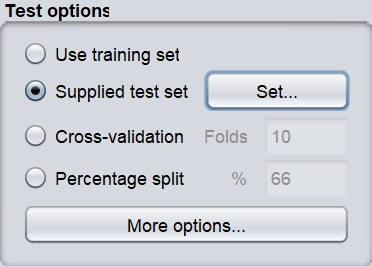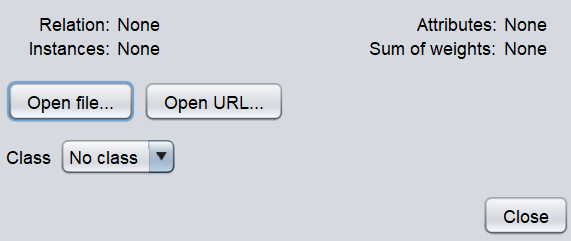This will open a pop-up window that will allow us to open the test instance file. It can be used to further increase the accuracy of the module by using different test sets.

My Personal Notes arrow_drop_up